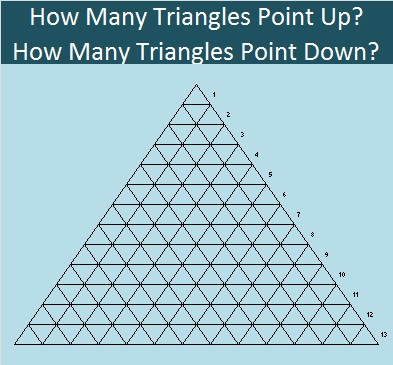# 658 How Many Triangles Point Up? How Many Triangles Point Down? How Many Triangles in All?When I first started counting the triangles in the figure, I started by counting the small triangles:

• In the top row there is 1 small triangle.
• In the top 2 rows there are 4 small triangles.
• In the top 3 rows there are 9 small triangles.
• In the top 4  rows there are 16 small triangles,
• and so forth so that in all 13 rows there are 169 small triangles.

As fascinating as that resulting squaring pattern is, it is NOT part of the most efficient way to count ALL the triangles of varying sizes.

The most efficient way to count ALL the triangles can be found on The University of Georgia’s website: Count the triangles that are pointing up separately from the triangles pointing down. Counting charts for triangles with 4, 5, 6, 7, and 8 rows of triangles are displayed on that website. I made a similar chart for these 13 rows of triangles:Notice all the triangular numbers on the chart!

Because 13 divided by 2 is 6.5, we see that 6 is the largest base size that has any triangles pointing down. Because 6.5 is not a whole number, there are 3 triangles that point down with a base size of 6.

Triangles made from an odd number of rows use these triangular numbers to count the triangles pointing down: 3, 10, 21, 36, 55, 78, etc.

Triangles made from an even number of rows have no remainder when divided by 2 so the triangle with the largest base size is the number of rows divided by two. There will only be one triangle with that base size and the triangular numbers used for that base size and smaller are 1, 6, 15, 28, 45, 66, 91, etc.

The Mathematics Stack Exchange had a discussion on how to count all the triangles, and a formula was posted:

The total number of triangles = ⌊n(n+2)(2n+1)/8⌋ Note: the brackets mean round decimals DOWN to the closest integer.

I made a chart showing the results of using the formula for n = 1 to 13:Thus we see that 658 is the total number of triangles that can be counted in a triangle made from 13 rows of triangles.

• 658 is a composite number.
• Prime factorization: 658 = 2 x 7 x 47
• The exponents in the prime factorization are 1, 1, and 1. Adding one to each and multiplying we get (1 + 1)(1 + 1)(1 + 1) = 2 x 2 x 2 = 8. Therefore 658 has exactly 8 factors.
• Factors of 658: 1, 2, 7, 14, 47, 94, 329, 658
• Factor pairs: 658 = 1 x 658, 2 x 329, 7 x 94, or 14 x 47
• 658 has no square factors that allow its square root to be simplified. √658 ≈ 25.65151.## 3 thoughts on “658 How Many Triangles Point Up? How Many Triangles Point Down? How Many Triangles in All?”

1.wbhs62

I’ve often mused about this, without ever getting stuck in to find out what the underlying situation is – thanks for the explanation.

•ivasallay

You’re welcome. I enjoyed learning about it, too.

This site uses Akismet to reduce spam. Learn how your comment data is processed.